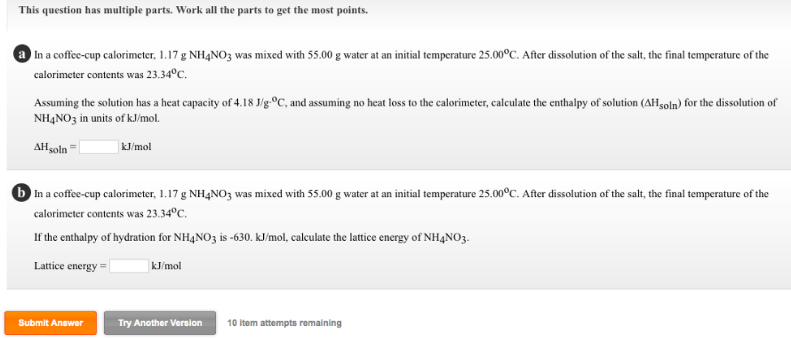# Problem: This question has multiple parts. Work all the parts to get the most points. In a coffee-cup calorimeter, 1.17 g NH4NO3 was mixed with 55.00 g water at an initial temperature 25.00°C. After dissolution of the salt, the final temperature of the calorimeter contents was 23.34°C. (a) Assuming the solution has a heat capacity of 4.18 J/g •°C, and assuming no heat loss to the calorimeter, calculate the enthalpy of solution (ΔHsoln) for the dissolution of NH4NO3 in units of kJ/mol. (b) In a coffee-cup calorimeter, 1.17 g NH4NO3 was mixed with 55.00 g water at an initial temperature 25.00°C. After dissolution of the salt, the final temperature of the calorimeter contents was 23.34°C. If the enthalpy of hydration for NH4NO3 is -630. kJ/mol, calculate the lattice energy of NH4NO3.

###### FREE Expert Solution
96% (351 ratings)###### Problem Details

This question has multiple parts. Work all the parts to get the most points.

In a coffee-cup calorimeter, 1.17 g NH4NO3 was mixed with 55.00 g water at an initial temperature 25.00°C. After dissolution of the salt, the final temperature of the calorimeter contents was 23.34°C.

(a) Assuming the solution has a heat capacity of 4.18 J/g •°C, and assuming no heat loss to the calorimeter, calculate the enthalpy of solution (ΔHsoln) for the dissolution of NH4NO3 in units of kJ/mol.

(b) In a coffee-cup calorimeter, 1.17 g NH4NO3 was mixed with 55.00 g water at an initial temperature 25.00°C. After dissolution of the salt, the final temperature of the calorimeter contents was 23.34°C.

If the enthalpy of hydration for NH4NO3 is -630. kJ/mol, calculate the lattice energy of NH4NO3